Next: Conclusions Up: Mathematical Properties of the Previous: Consequences   Contents

# Weighted Average

Suppose each element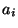has a corresponding weight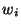. This weight' is essentially how important the particular number is. A number with no importance has a weight of 0. To give one number twice the importance of another, give it twice the weight. The typical example: suppose you took a test (got 90) and a quiz (got an 80). Tests are twice as important as quizes. How would you take the average? One way to do it is to count the test as if we took it twice, and got 90 both times. After all, it is twice as important, and gets twice as many votes'. So,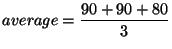However, let's use the weight method. Give the test a weight of 2, and the quiz a weight of 1. Thus, there are 3 total votes (This is important: the denominator is not the number of elements: it is the sum of all the weights, or votes. Previously, each element got one vote, so denominator = number of votes = number of elements).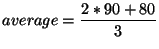In general, the formula is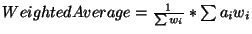This looks worse than it is. Instead of dividing by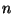, we divide by the sum of the weights. Instead of adding updirectly, we multiply eachby, then add them up. Hey, wouldn't it be cool if the sum of the weights was 1? Then we wouldn't have to do any division at the end. This is a commonly used tactic: make eachrepresent the percentage of the total weight. The sum of all the percentages is 1, so no division is required. This is often used in academic settings (i.e., tests count 50%, quizes 30%, and homework 20%). Another use is when each element is different for some reason or another. In the center of gravity example, each weight did not have to be 1-pound. Instead, have the distance of each weight beand the mass be. The weighted average is the center of gravity; this is how the calculations are actually done (I will put up an article about this someday).Next: Conclusions Up: Mathematical Properties of the Previous: Consequences   Contents